# Periodicity In Properties -1 - Periodic Properties Chemistry Notes | EduRev

## Chemistry : Periodicity In Properties -1 - Periodic Properties Chemistry Notes | EduRev

The document Periodicity In Properties -1 - Periodic Properties Chemistry Notes | EduRev is a part of the Chemistry Course Inorganic Chemistry.
All you need of Chemistry at this link: Chemistry

PERIODICITY IN PROPERTIES

The periodicity is repetition of elements with similar properties after certain regular intervals when the elements are arranged in order of increasing atomic number.
The periodic repetition of properties is due to the recurrence of similar valence shell configurations after regular intervals.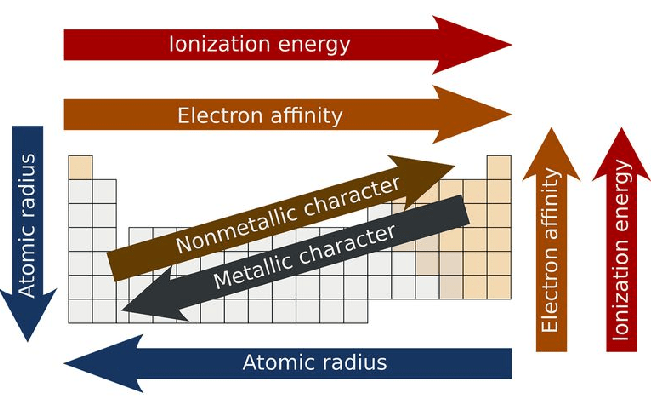Periodicity in periodic table

Atomic Size

Factors affecting atomic size
(a) n increase size increases (n = principal of quantum number)
(b) Zeff increase, size decrease [Zeff = Z – σ]

Periodic Trends

In a period (L → R) :  Atomic size decreases from left to right in a period.
Looking to the E.C. of elements, following observations are made,

• The e-s enter in the same shell,
• The no. of protons are increasing from left to right with increase in atomic no.
• The nuclear charge is increasing, so the attractive force of nucleus on outermost e is increasing.

This results in net contraction of atomic size.
In a group (up to down): Atomic size increases from top to down in a group.
The electronic configuration of elements of the same group indicates that e-s are added in the new shell in the senior atom. i.e. a new shell is added in the next higher group.

• Although no. of protons are also increasing (i.e. increase in nuclear charge) but the net result is increase in size of the atom.
• The net attractive force of nucleus decreases down the group.

An atom does not have any sharp boundary,

• So the atomic radius is determined by indirect method in the combined state of an atom.

The atomic radii of atoms of compounds are classified as :

(i) Covalent radii (in covalent molecules),
(ii) Ionic radii (in ionic crystals)
(iii) Van-der-wall radii (in atomic crystals)

Variation in atomic radius in a group :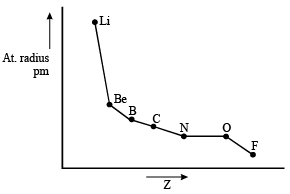The distance between the nucleus and the outer most shell of an ion is known as ionic radii.

• A cation is smaller than parent atom and an anion is larger than parent atom.
 Cationic Radii (in Å) Na(1.54)  & Na+(.95) Mg(1.36) & Mg+2(.65) Anionic Radii F(.72)  & F–(1.36) Cl(.99) & Cl–(1.81)

Size of isoelectronic ions:

• Isoelectronic ions have the same number of electrons
• Al3+, Mg2+, Na+, Ne, F, O2 and N3 all have 10 electrons
⇒ All have the same configuration : 1s2 2s2 2p6 (which is the noble gas : Neon)
⇒ Positive ions that have more protons would be smaller (more protons would pull the same number of electrons)
• For  isoelectronic species Size depends upon Z, more Z less size

Size
(i) C+4 < B+3 < Be2+ < Li+
(ii)  Al3+ < Mg2+ < Na+ < Ne < F < O2– < N3–

Example: Compare size of the following
(i) S2- , Cl- , K+  , Ca 2+ , Sc 2+
(ii) SO3 , NO3- , CO32- , COCl2
(iii) N2 , CO , CN-
(iv) NH3 , H3O+
(v) H- , He , Li+
(vi) CH4 , NH4+
(vii) NCS- , CS2

Ionisation Energy Amount of energy required to remove the most loosely bounded electron from an isolated gaseous atom.

Ionisation is endothermic (endoergic) i.e. requires energy hence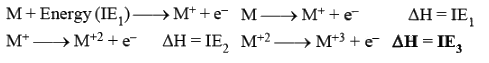• IE3 > IE2 > IE1 always
•  Ionization energy is also called Ionization Potential (l.P.).

Unit: lonisation energy is expressed in the units of energy, i.e. (ev) per atom;  (kJ mol-1);  kcal mol-1
1 eV/atom = 1.602 ´ 10-16 kJ / atom = 1.602 x 10-16 x 6.02 x 1023 kJ / mol = 96.4 x10kJmol -1

Factors Affecting I.E. :

(i) Size of Atom: Varies inversely

• As the size of atom increases.  The outer most electrons are less tightly held by the nucleus.
• As a result it is easier to remove the e-.
• The ionisation energy decreases with increase in atomic size.

(ii) Nuclear Charge: Varies directly

• As nuclear charge increases, it becomes difficult to remove the e-, so ionisation energy increases.

(iii) Screening Effect: Varies inversely

(iv) Nature of orbitals containing e-s: (Penetrating effect of e-s)

(v) Electronic Configuration (E.C.) : The atoms having stable E.C. have less tendency to lose es. So have high I.E.

(a) Half filled E.C.:   N = 1s2, 2s2, 2p

(b) Full filled E.C. (Noble gas configuration):   Ne = 1s2, 2s2, 2p6

(c) E.C. with all e-s paired: E.g. Be = 1s2, 2s2;    Zn = 3d104s2;    Cd = 4d10 5s2;  Hg = 5d106s2

General Trend Across the Period: L → R .
Along period I.E. increases [with some exception] [Zeff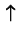­]

Reason: (a)  Nuclear Charge ­(b)  Atomic Size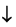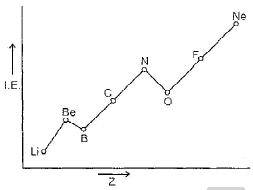Example: Explain that

(i) Li < Be,   (ii) Be > B,  (iii) B < C < N,  (iv) N > O,  (v)  O < F < Ne

In a Group :  Along a group I.E. decrease [Zeff constant, n­ ]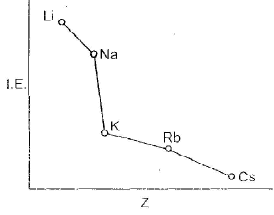Reason :

(a) Atomic size increases

(b) Increase in screening effect of electrons of inner shells also increase which nullify the increased Nuclear charge.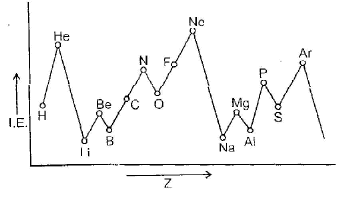Fig. : First ionisation energies of the elements

Conclusion
1.
Maxima and minima occur at the noble gases and alkali metals respectively indicating special stability associated with closed shell configuration of noble gases.
2. The variation in I.E. along a series of transition metal or inner transition element is much less than that along a period of non transition elements.
3. Half filled E.C. have higher I.E. than the next element along a period (N > O) (P > S).

• Determination of I.E. : I.E. is the difference between energy of a gaseous atom and that of the system, gaseous ion + free e.
(i) Li < B< Be<C <O < N < F < Ne
(ii) Na < Al < Mg < Si < S < P < Cl < Ar
(iii) K < Ga < Ca < Ge < Se < As < Br < Kr
(iv) Rb < Sr < In < Sn < Sb < Te < I < Xe
(v) Cs < Ba < Tl < Bi < Pb < Po < At < Rn

I.E. variation in transition metals with dn E.C.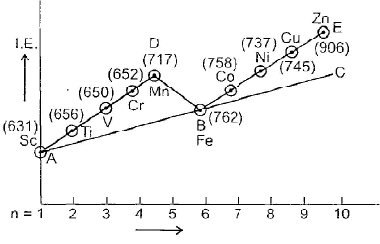Exception
(1) Along a period, half filled and fully filled have higher I.E.
e.g. Be > B and N > O.
(2) along a group, Ga > Al

Properties affected by Ionisation Energy:

(1) Metallic character : More ionisation energy means less metallic character (in a period).

(2) Tending to stay in which state A+1, A+2 or A+3 is stable.

Offer running on EduRev: Apply code STAYHOME200 to get INR 200 off on our premium plan EduRev Infinity!

## Inorganic Chemistry

37 videos|38 docs|18 tests

,

,

,

,

,

,

,

,

,

,

,

,

,

,

,

,

,

,

,

,

,

;